# Overview: PROBIT Procedure

The PROBIT procedure calculates maximum likelihood estimates of regression parameters and the natural (or threshold) response rate for quantal response data from biological assays or other discrete event data. This includes probit, logit, ordinal logistic, and extreme value (or gompit) regression models.

Probit analysis developed from the need to analyze qualitative (dichotomous or polytomous) dependent variables within the regression framework. Many response variables are binary by nature (yes/no), while others are measured ordinally rather than continuously (degree of severity). Collett (2003) and Agresti (2002), for example, have shown ordinary least squares (OLS) regression to be inadequate when the dependent variable is discrete. Probit or logit analyses are more appropriate in this case.

The PROBIT procedure computes maximum likelihood estimates of the parametersand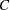of the probit equation by using a modified Newton-Raphson algorithm. When the response Y is binary, with values 0 and 1, the probit equation is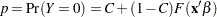whereis a vector of parameter estimatesis a cumulative distribution function (normal, logistic, or extreme value)is a vector of explanatory variablesis the probability of a responseis the natural (threshold) response rate

Notice that PROC PROBIT, by default, models the probability of the lower response levels. The choice of the distribution function(normal for the probit model, logistic for the logit model, and extreme value or Gompertz for the gompit model) determines the type of analysis. For most problems, there is relatively little difference between the normal and logistic specifications of the model. Both distributions are symmetric about the value zero. The extreme value (or Gompertz) distribution, however, is not symmetric, approaching 0 on the left more slowly than it approaches 1 on the right. You can use the extreme value distribution where such asymmetry is appropriate.

For ordinal response models, the response, Y, of an individual or an experimental unit can be restricted to one of a (usually small) number,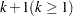, of ordinal values, denoted for convenience by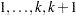. For example, the severity of coronary disease can be classified into three response categories as 1=no disease, 2=angina pectoris, and 3=myocardial infraction. The PROBIT procedure fits a common slopes cumulative model, which is a parallel-lines regression model based on the cumulative probabilities of the response categories rather than on their individual probabilities. The cumulative model has the form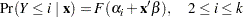where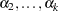are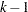intercept parameters. By default, the covariate vectorcontains an overall intercept term.

You can set or estimate the natural (threshold) response rate. Estimation ofcan begin either from an initial value that you specify or from the rate observed in a control group. By default, the natural response rate is fixed at zero.

An observation in the data set analyzed by the PROBIT procedure might contain the response and explanatory values for one subject. Alternatively, it might provide the number of observed events from a number of subjects at a particular setting of the explanatory variables. In this case, PROC PROBIT models the probability of an event.

The PROBIT procedure uses ODS Graphics to create graphs as part of its output. For general information about ODS Graphics, see Chapter 21, Statistical Graphics Using ODS. For specific information about the graphics available in the PROBIT procedure, see the section ODS Graphics.